Скачать презентацию Universal Weight Function Plan Part I Weight functions

c8f28d451a05262f06bf50eee6991ba1.ppt

• Количество слайдов: 21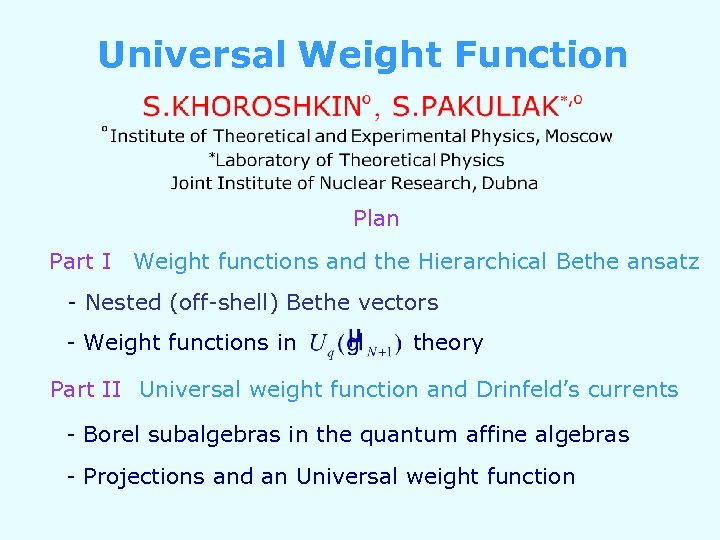Universal Weight Function Plan Part I Weight functions and the Hierarchical Bethe ansatz - Nested (off-shell) Bethe vectors - Weight functions in theory Part II Universal weight function and Drinfeld’s currents - Borel subalgebras in the quantum affine algebras - Projections and an Universal weight function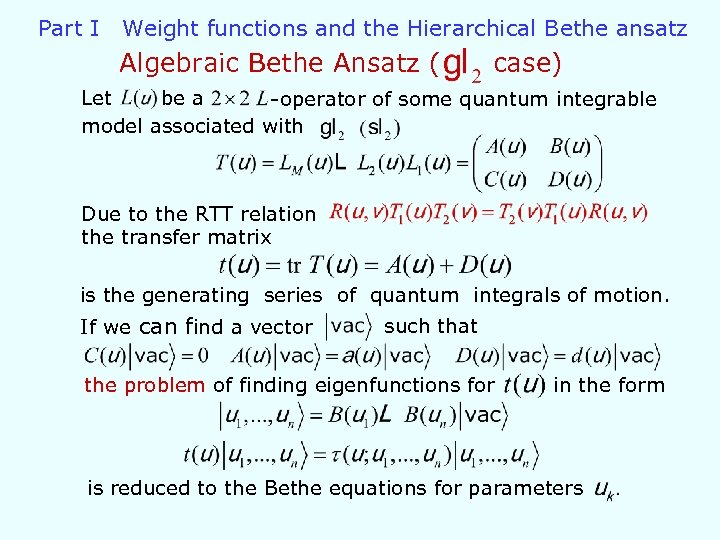Part I Weight functions and the Hierarchical Bethe ansatz Algebraic Bethe Ansatz ( case) Let be a -operator of some quantum integrable model associated with Due to the RTT relation the transfer matrix is the generating series of quantum integrals of motion. If we can find a vector such that the problem of finding eigenfunctions for in the form is reduced to the Bethe equations for parameters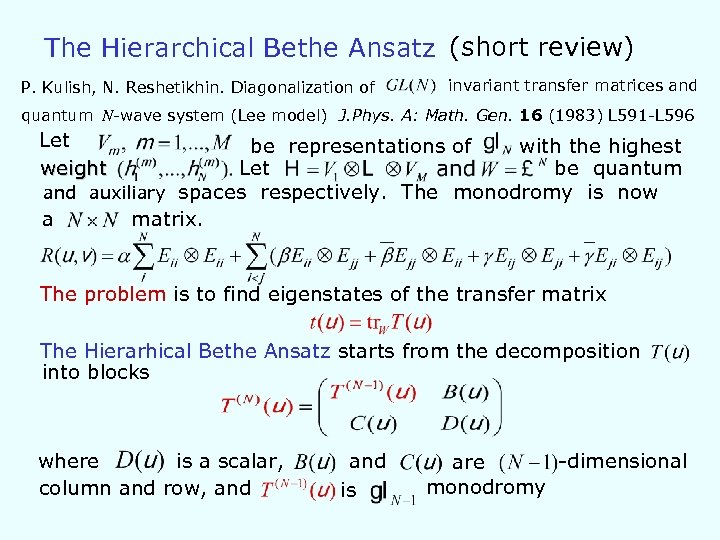The Hierarchical Bethe Ansatz (short review) P. Kulish, N. Reshetikhin. Diagonalization of invariant transfer matrices and quantum N-wave system (Lee model) J. Phys. A: Math. Gen. 16 (1983) L 591 -L 596 Let weight be representations of with the highest Let be quantum and auxiliary spaces respectively. The monodromy is now a matrix. The problem is to find eigenstates of the transfer matrix The Hierarhical Bethe Ansatz starts from the decomposition into blocks where is a scalar, column and row, and is -dimensional are monodromy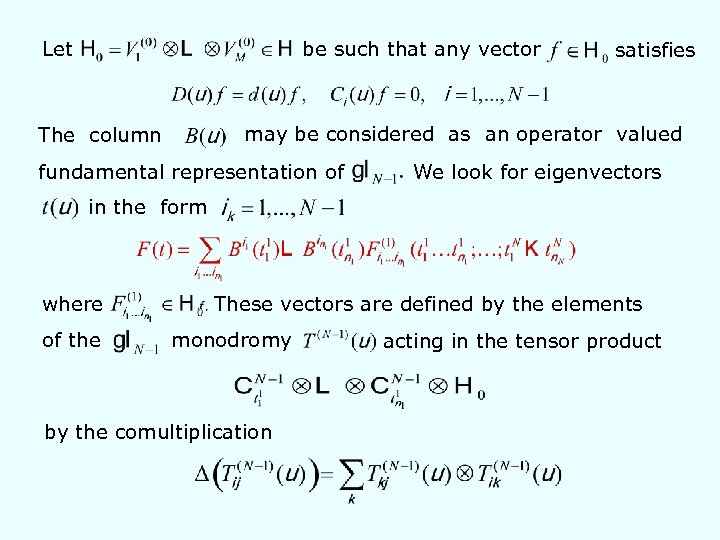Let be such that any vector satisfies may be considered as an operator valued The column fundamental representation of We look for eigenvectors in the form where of the These vectors are defined by the elements monodromy by the comultiplication acting in the tensor product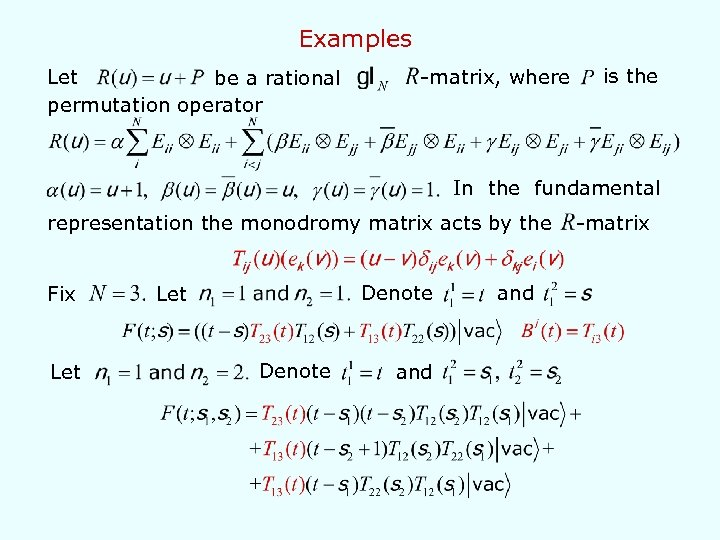Examples Let be a rational permutation operator -matrix, where is the In the fundamental representation the monodromy matrix acts by the Fix Let Denote and -matrix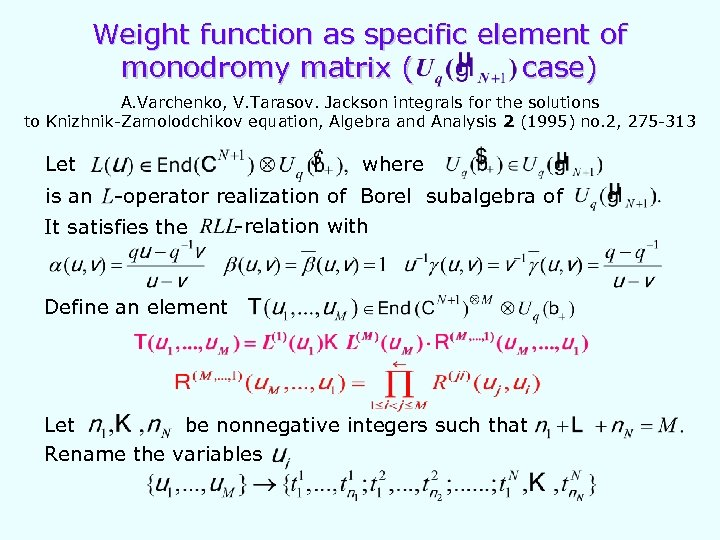Weight function as specific element of monodromy matrix ( case) A. Varchenko, V. Tarasov. Jackson integrals for the solutions to Knizhnik-Zamolodchikov equation, Algebra and Analysis 2 (1995) no. 2, 275 -313 Let is an where -operator realization of Borel subalgebra of It satisfies the -relation with Define an element Let be nonnegative integers such that Rename the variables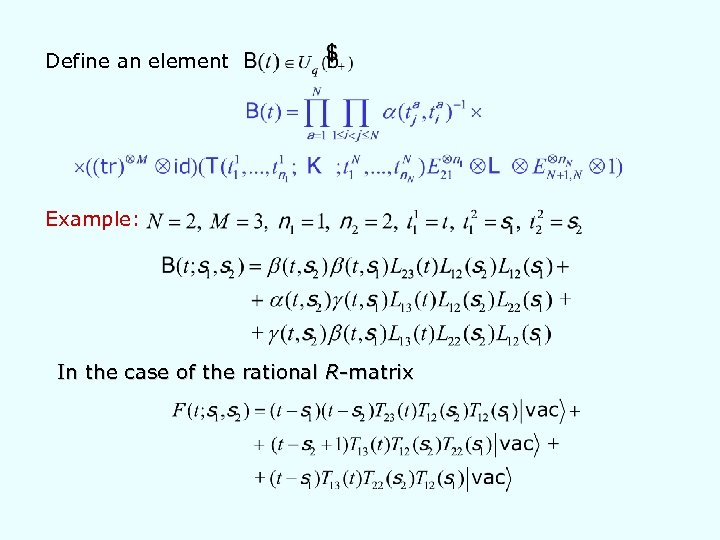Define an element Example: In the case of the rational R-matrix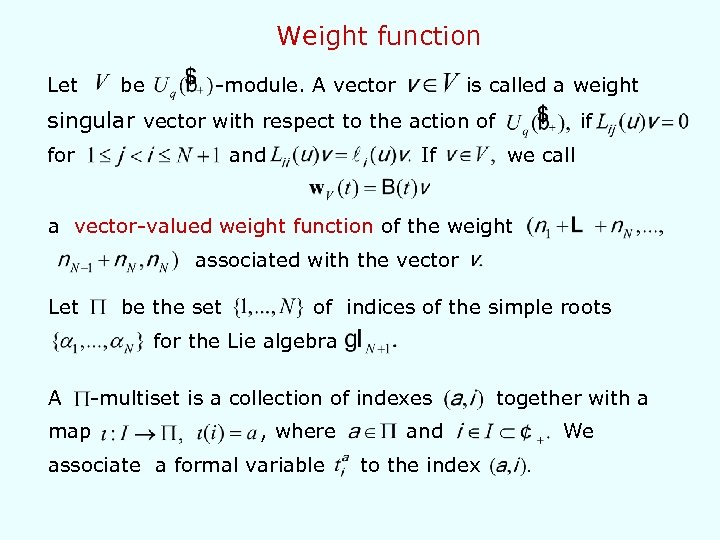Weight function Let be -module. A vector is called a weight singular vector with respect to the action of for and If if we call a vector-valued weight function of the weight associated with the vector Let be the set of indices of the simple roots for the Lie algebra A -multiset is a collection of indexes map , where associate a formal variable and to the index together with a We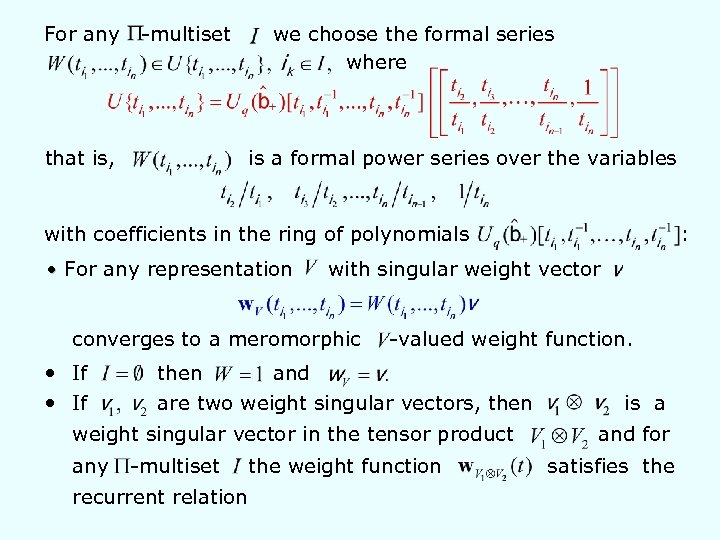For any -multiset that is, we choose the formal series where is a formal power series over the variables with coefficients in the ring of polynomials • For any representation with singular weight vector converges to a meromorphic • If -valued weight function. then and are two weight singular vectors, then weight singular vector in the tensor product any -multiset recurrent relation : the weight function is a and for satisfies the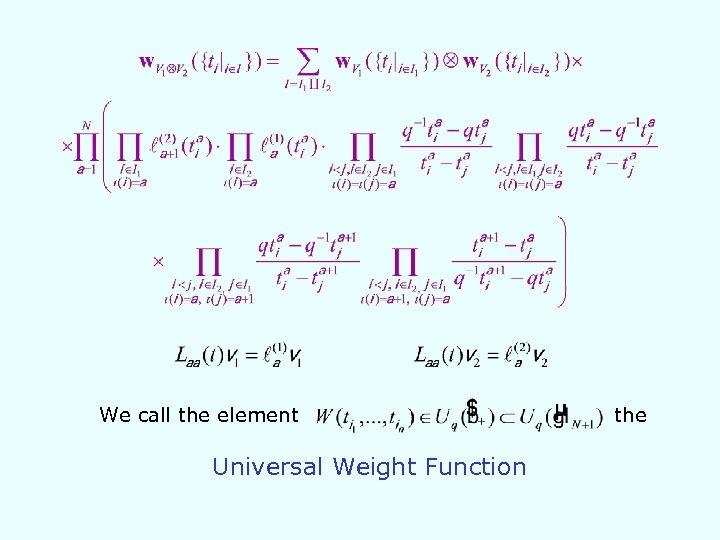We call the element Universal Weight Function the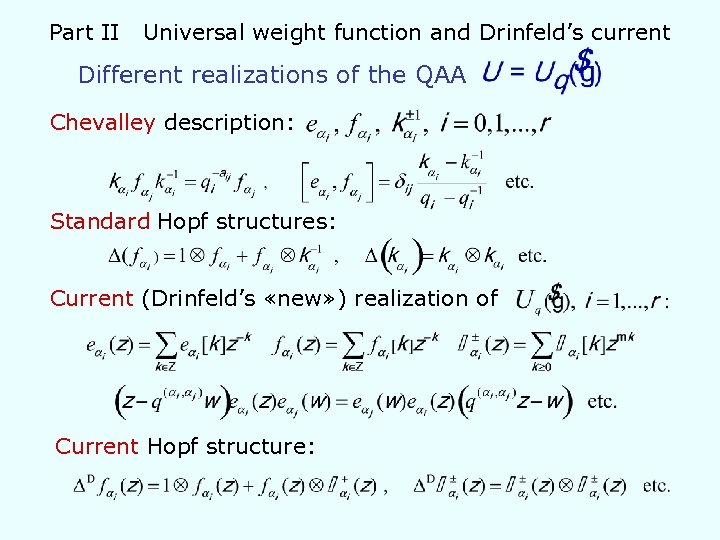Part II Universal weight function and Drinfeld’s current Different realizations of the QAA Chevalley description: Standard Hopf structures: Current (Drinfeld’s «new» ) realization of Current Hopf structure: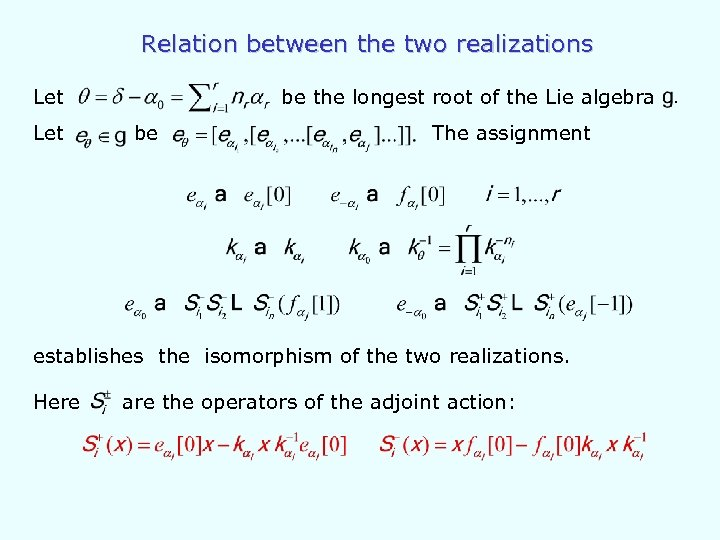Relation between the two realizations Let be the longest root of the Lie algebra be The assignment establishes the isomorphism of the two realizations. Here are the operators of the adjoint action: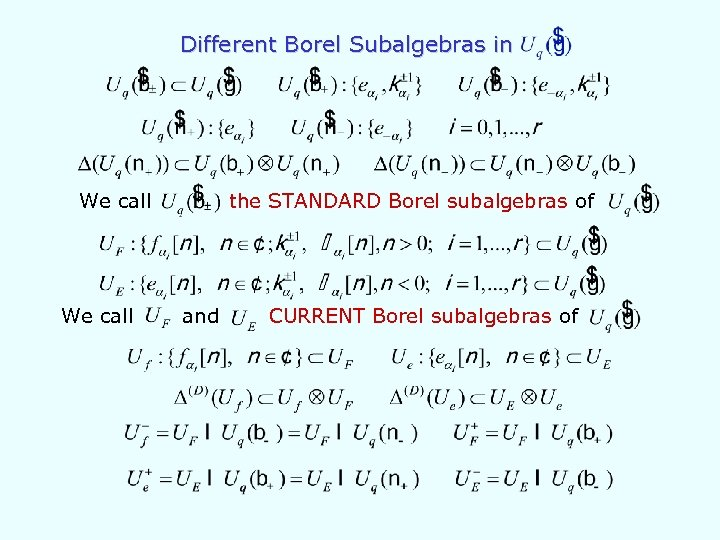Different Borel Subalgebras in We call the STANDARD Borel subalgebras of and CURRENT Borel subalgebras of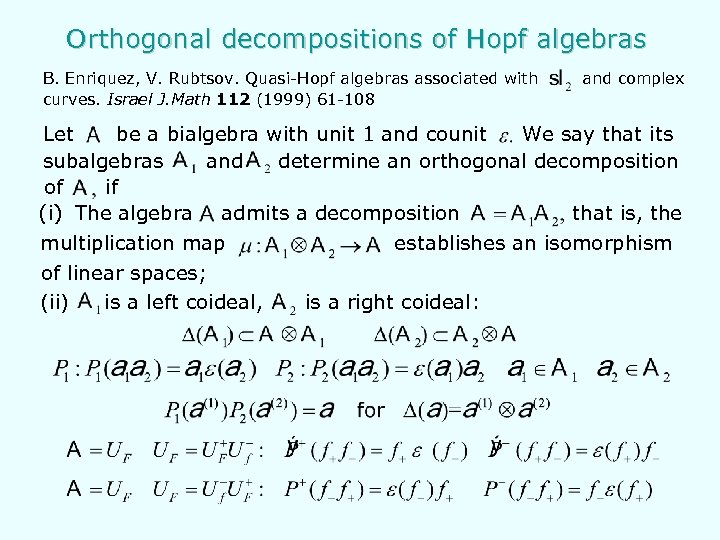Orthogonal decompositions of Hopf algebras B. Enriquez, V. Rubtsov. Quasi-Hopf algebras associated with curves. Israel J. Math 112 (1999) 61 -108 and complex Let be a bialgebra with unit 1 and counit We say that its subalgebras and determine an orthogonal decomposition of if (i) The algebra admits a decomposition that is, the multiplication map of linear spaces; (ii) is a left coideal, establishes an isomorphism is a right coideal: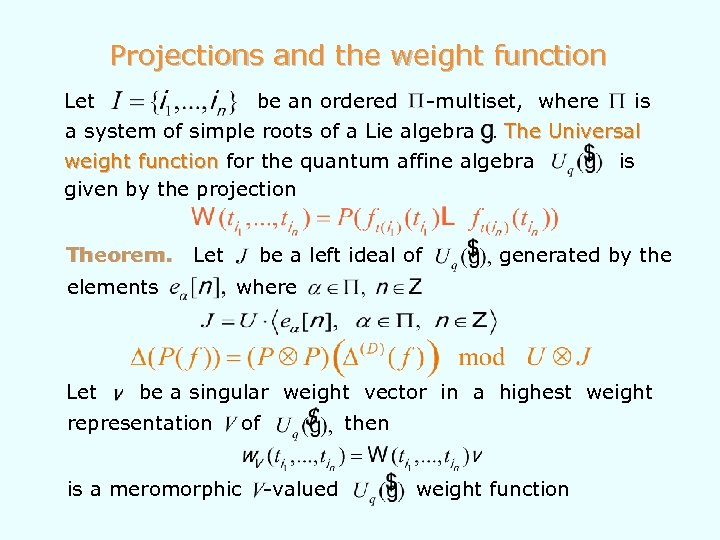Projections and the weight function Let be an ordered -multiset, where is a system of simple roots of a Lie algebra The Universal weight function for the quantum affine algebra is given by the projection Theorem. Let elements Let be a left ideal of generated by the where be a singular weight vector in a highest weight representation of is a meromorphic then -valued weight function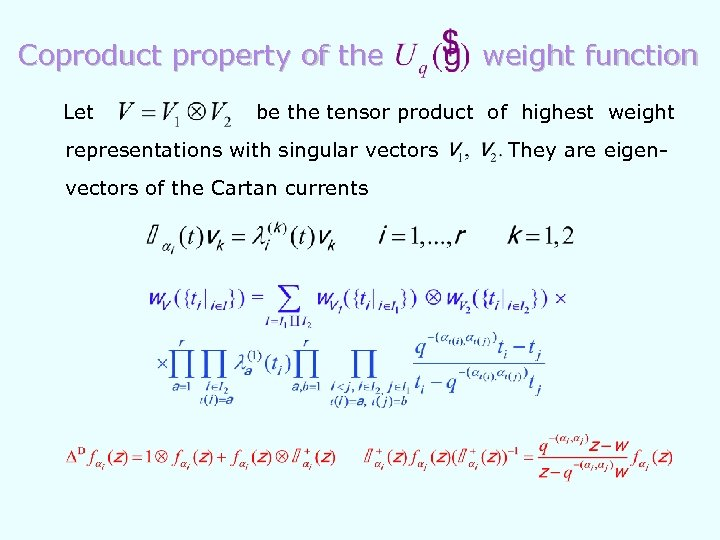Coproduct property of the Let weight function be the tensor product of highest weight representations with singular vectors of the Cartan currents They are eigen-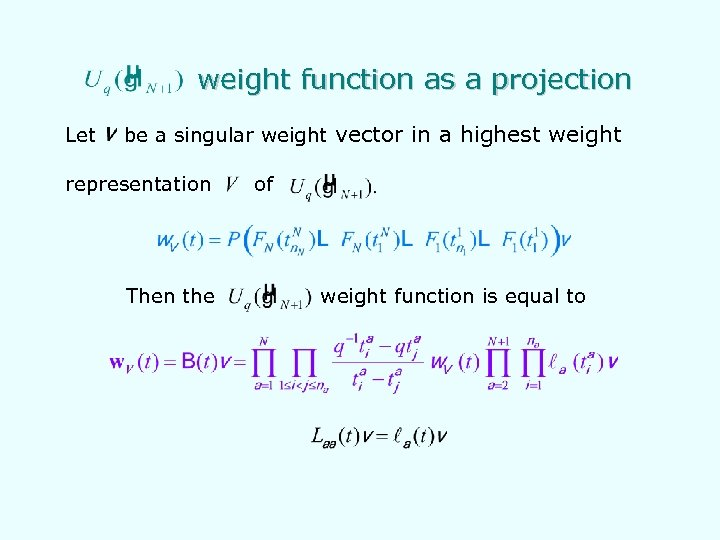weight function as a projection Let be a singular weight vector in a highest weight representation Then the of weight function is equal to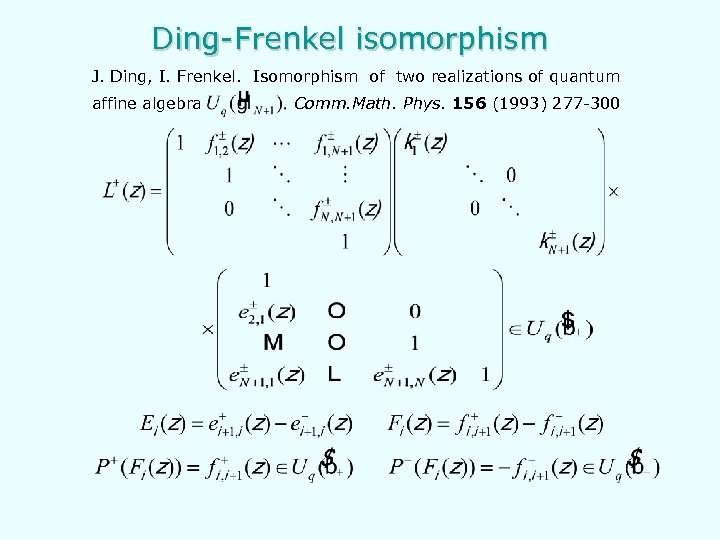Ding-Frenkel isomorphism J. Ding, I. Frenkel. Isomorphism of two realizations of quantum affine algebra Comm. Math. Phys. 156 (1993) 277 -300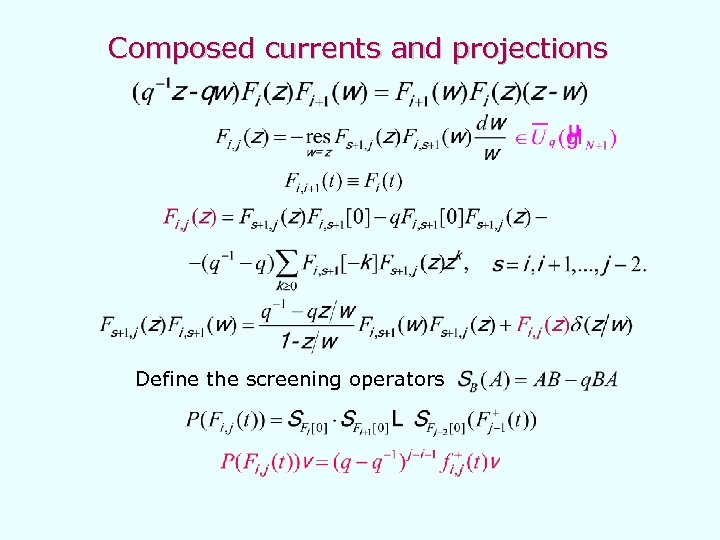Composed currents and projections Define the screening operators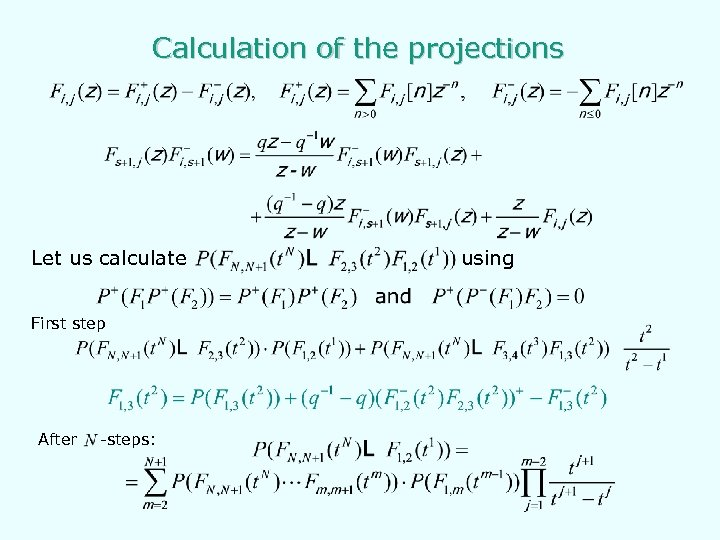Calculation of the projections Let us calculate First step After -steps: using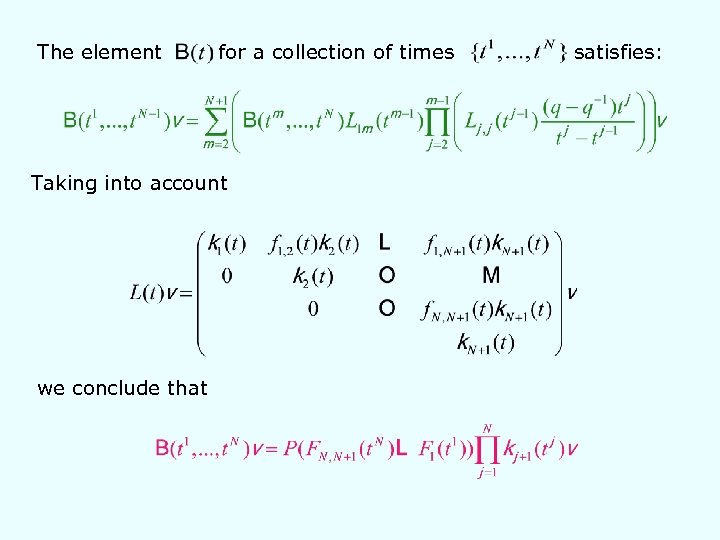The element for a collection of times Taking into account we conclude that satisfies: